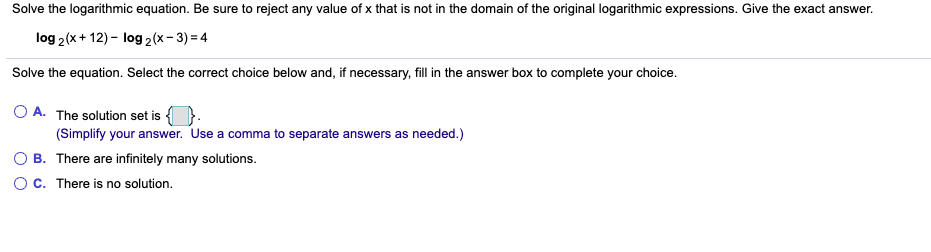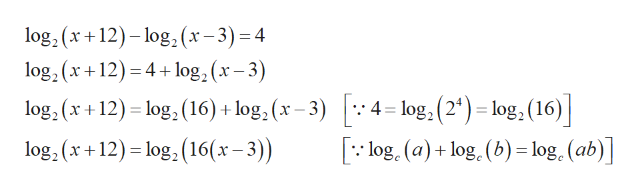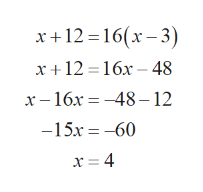# Solve the logarithmic equation. Be sure to reject any value of x that is not in the domain of the original logarithmic expressions. Give the exact answer.log 2(x+ 12) - log 2(x- 3) = 4Solve the equation. Select the correct choice below and, if necessary, fill in the answer box to complete your choice.O A. The solution set is {(Simplify your answer. Use a comma to separate answers as needed.)B. There are infinitely many solutions.C. There is no solution.

Question
1 viewshelp_outlineImage TranscriptioncloseSolve the logarithmic equation. Be sure to reject any value of x that is not in the domain of the original logarithmic expressions. Give the exact answer. log 2(x+ 12) - log 2(x- 3) = 4 Solve the equation. Select the correct choice below and, if necessary, fill in the answer box to complete your choice. O A. The solution set is { (Simplify your answer. Use a comma to separate answers as needed.) B. There are infinitely many solutions. C. There is no solution. fullscreen
check_circle

Step 1

Given the logarithmic equation

Step 2

Simplify as follows.help_outlineImage Transcriptioncloselog, (x +12)– log,2(x- 3)=4 log, (x+12) = 4 + log, (x – 3) %3D log, (x +12) = log, (16) + log, (x – 3) |: 4= log, (2') = log, (16) | [: log. (a) + log. (b) = log. (ab)] log, (x +12)= log, (16(x – 3)) %3D fullscreen
Step 3

Using one-to-one property o...help_outlineImage Transcriptionclosex+12 =16(x– 3) x+12 = 16x – 48 х—16х — —48-12 -15x = -60 х fullscreen

### Want to see the full answer?

See Solution

#### Want to see this answer and more?

Solutions are written by subject experts who are available 24/7. Questions are typically answered within 1 hour.*

See Solution
*Response times may vary by subject and question.
Tagged in

### Logarithms